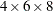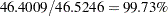The OPTEX Procedure

## Example 10.2 Comparing Fedorov Algorithm to Sequential Algorithm

[See OPTEX4 in the SAS/QC Sample Library]An automotive engineer wants to fit a quadratic model to fuel consumption data in order to find the values of the control variables that minimize fuel consumption (refer to Vance 1986). The three control variables AFR (air fuel ratio), EGR (exhaust gas recirculation), and SA (spark advance) and their possible settings are shown in the following table:

Variable

Values

AFR

15

16

17

18

EGR

0.020

0.177

0.377

0.566

0.921

1.117

SA

10

16

22

28

34

40

46

52

Rather than run all 192 () combinations of these factors, the engineer would like to see whether the total number of runs can be reduced to 50 in an optimal fashion.

Since the factors have different numbers of levels, you can use the PLAN procedure (refer to the SAS/STAT 9.22 User's Guide) to generate the full factorial set to serve as a candidate data set for the OPTEX procedure.

```   proc plan;
factors AFR=4 ordered EGR=6 ordered SA=8 ordered
/ noprint;
output out=a
AFR nvals=(15, 16, 17, 18)
EGR nvals=(0.020, 0.177, 0.377, 0.566, 0.921, 1.117)
SA  nvals=(10, 16, 22, 28, 34, 40, 46, 52);
run;
```

The Fedorov algorithm (Fedorov 1972) is generally the most successful optimal design search algorithm, although it also typically can take relatively much longer to run than other algorithms. This algorithm is not the default search method for the OPTEX procedure. However, you can specify that it be used with the METHOD=FEDOROV option in the GENERATE statement. For example, the following statements produce Output 10.2.1.

```proc optex data=a seed=61552;
model AFR|EGR|SA@2 AFR*AFR EGR*EGR SA*SA;
generate n=50 method=fedorov iter=100 keep=10;
run;
```

Output 10.2.1 Efficiencies with Fedorov Algorithm
The OPTEX Procedure

Design Number D-Efficiency A-Efficiency G-Efficiency Average Prediction
Standard Error
1 46.5246 24.5897 96.3915 0.4231
2 46.5241 24.5901 96.3926 0.4233
3 46.5238 24.5844 96.2306 0.4231
4 46.5237 24.5855 96.2318 0.4233
5 46.5219 24.5866 96.4790 0.4233
6 46.5192 24.5832 96.3070 0.4231
7 46.5192 24.5832 96.3070 0.4231
8 46.5190 24.5741 96.1695 0.4232
9 46.5189 24.5841 96.3062 0.4233
10 46.5188 24.5755 96.3020 0.4234

The Fedorov search method for the preceding problem requires a few seconds for 100 tries on a 2.8GHz desktop PC.

For comparison, you can use the METHOD=SEQUENTIAL option in the GENERATE statement, as shown in the following statements, which produce Output 10.2.2.

```proc optex data=a seed=33805;
model AFR|EGR|SA@2 AFR*AFR EGR*EGR SA*SA;
generate n=50 method=sequential iter=100 keep=10;
run;
```

Output 10.2.2 Efficiencies with Sequential Algorithm
Design Number D-Efficiency A-Efficiency G-Efficiency Average Prediction
Standard Error
1 46.5246 24.5897 96.3915 0.4231
2 46.5241 24.5901 96.3926 0.4233
3 46.5238 24.5844 96.2306 0.4231
4 46.5237 24.5855 96.2318 0.4233
5 46.5219 24.5866 96.4790 0.4233
6 46.5192 24.5832 96.3070 0.4231
7 46.5192 24.5832 96.3070 0.4231
8 46.5190 24.5741 96.1695 0.4232
9 46.5189 24.5841 96.3062 0.4233
10 46.5188 24.5755 96.3020 0.4234

In a fraction of the run time required by the Fedorov method, the sequential algorithm finds a design with a relative D-efficiency ofcompared to the best design found by the Fedorov method, and with better A-efficiency. As this demonstrates, if absolute D-optimality is not required, a faster, simpler search may be sufficient.Previous Page | Next Page | Top of Page